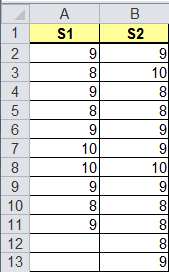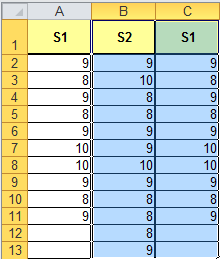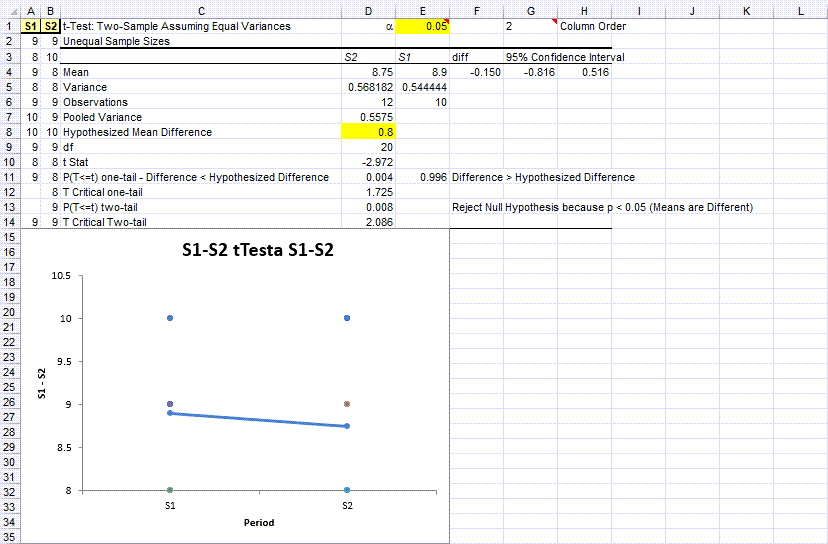# Two One-Sided Test (TOST) in Excel

## QI Macros Add-in Makes it Easy to Conduct a TOST Test in Excel

### When to use a Two One-Sided Test for Equivalence

While measurements at different times or using different formulations can vary, it's often useful to determine if the two measurements produce equivalent results. Traditional hypothesis testing seeks to determine if means are the same or different, but it can have false positives. Equivalence testing determines an interval where the means can be considered equivalent.

The Two One-Sided Test uses two t-tests assuming equal variances with a hypothesized mean difference (u1-u2 = interval).

Note: Excel does not do equivalence tests; QI Macros adds this functionality.

### Two One-Sided Test Excel Using QI Macros

Adhesive tape is measured immediately after production and 24 hours later. Are the measurements equivalent at these two different points in time? If they are, one measurement can be eliminated, saving time and money.

Define the two null and alternate Hypotheses for equivalence:

• The null hypothesis H01 is that the mean difference (x1-x2) <= 0.8
• The alternative hypothesis H11a is that the mean difference > 0.8
• The null hypothesis H02 is that the mean difference (x1-x2) >= 0.8
• The alternative hypothesis H12a is that the mean difference < 0.8

#### Run a t-Test Assuming Equal Variances of Sample 1 and 2Click and drag over the data to select it and then click on QI Macros Menu -> Statistical Tools -> F & Tests -> t-Test: Two sample assuming equal variances:QI Macros will prompt for a significance level (default = 0.05 or 0.95 depending on the order of S1 and S2) and hypothesized difference in the means:QI Macros t test two-sample macro will perform the calculations and interpret the results for you. The one-tailed p value of 0.028 < 0.05.### Repeat the t-Test, but reverse the order of S1 and S2:

Copy column A to column C, then select B1:C13.Click on QI Macros -> Statistical Tools -> F & t Tests -> t-Test: two-sample assuming equal variances:Answer the prompts with the same values.

The one-tailed p-value of 0.004 < 0.05.Now here's where it gets interesting...because the one-tailed p-values are both less than 0.05...
the means can be declared equivalent. So, we can eliminate one of the tests.

### Why Choose QI Macros Statistical Software for Excel?### Affordable

• Only \$329 USD - less with quantity discounts
• No annual fees
• Free Technical Support### Easy to Use

• Works Right in Excel
• Interprets Results for You
• Accurate Results Without Worry### Proven and Trusted

• 100,000 Users in 80 Countries
• Celebrating 20th Anniversary
• Five Star CNET Rating - Virus Free